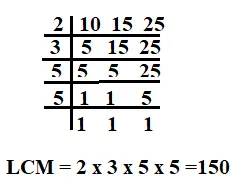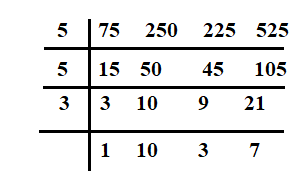December 8, 2023

Are you a Class 5 student looking to improve your understanding of the Least Common Multiple (LCM)? Look no further! In this post ” LCM sums for class 5 with answers” ,  we will explore LCM sums specifically designed for Class 5 students. By practicing these problems, you’ll enhance your LCM skills and gain confidence in solving related questions.

By solving these LCM sums, you will improve your mathematical reasoning and problem-solving skills. The more you practice, the better you will become at identifying the least common multiple of various numbers.

Contents

# LCM Sums for Class 5 with answers (Solved examples)

Example 1: Find the LCM of 4 and 6 by finding their common multiples.

Solution:  The multiples of 4 are 4, 8, 12, 16, 20, 24, 28, ………

The multiples of 6 are 6, 12, 18, 24, 30, 36, ………

The common multiples of 4 and 6 are 12, 24, 36, ………..

Hence, the LCM of 4 and  6 = 12

Example 2:  Find the LCM of  28 and 30 by the prime factorization method .

Solution. Step 1 Write the prime factors of 28 and 30 .

Step 2. Circle the common factors

Step 3. Multiply the common factors (only once) and the factors that are not common.

Hence the LCM of 28 and 30 is 420.

Example 3 : Find the LCM of 8 and 12 by short division method.

Solution.  Step 1. Divide the numbers by their smallest common prime factor and write the quotient below them. If a number cannot be divided exactly , copy the number.

Step 2. Continue  dividing by common prime factors writing the quotient below each number.

Step 3. Stop when there is no common prime factor.

Hence the LCM of 8 and 12 is 24.

Example 4 : What is the least number of children that can be arranged in rows of 10, 15 or 25 children in each row?

Solution.  The least number of children that cab be arranged in rows will be the LCM of 10, 15 and 25.The least number of children that can be arranged in rows is 150.

Example 5.  Five bells commence tolling together and toll at intervals of  6, 7,  8,  and 12 seconds respectively. After how much time will they toll together again?

Ans. Required time = LCM of 6, 7, 8 and 12

= 168 seconds

So , all the bells will toll together after  168 seconds  or 2 min. 48 sec.

Example 6: The HCF of two numbers is 144 and their LCM is 2880. If one of the numbers is 720, Find the other number.

Solution :For any two given numbers, we have  First number x second number = Their HCF x Their LCM

HCF= 144 ,  LCM = 2880  and one number = 720

The other number=

hence , the other number is =576.

Example 7: Find the LCM of 75, 250, 225 and 525 by division method.

Solution. We have,LCM of given numbers = 5 x 5  x 3 x 10 x 3 x 7 = 15750

## LCM Sums for Class 5 with answers(Unsolved)

A. Fill in the blanks –

1)  The  …………. of two or more numbers is the smallest number that is completely divisible by each of the numbers.

2)  The LCM of two or more numbers cannot be  ……………….. the numbers themselves.

3) If one number is  a factor of other , the ……………..  number is the LCM.

4) The LCM of co-prime numbers is their ………….

5) Product of two given numbers is equal to the product of their HCF and  ……….

B. Solve the following.

1) Find the LCM of 10, 15 and 18 by the prime factorization method.

2) Find the LCM of 21, 14 and 42 by Short division method.

3)  Find the LCM of  10, 15 and 18.

4) Find the LCM of 48 and 72 by prime factorization method.

5) Find the LCM of 90, 108 and 144 by prime factorization method.

6)Find the LCM of 20 ,  30  and 50 by short division method.

7)Find the LCM of 12, 18 , 24 and 36 by short division method.

8) Find the LCM of 96, 108 , 24 and 180 by short division method.

9) Six bells commence tolling together and toll at intervals of 2,4 , 6, 8, 10 and 12 seconds respectively. After how much time will they toll together again?

10)  Find the least number which is exactly divisible by each one of the numbers 12, 16  and 24.

11) Find the least number of stones so that heaps of 15, 20 and 30 stones can be made.

12) The HCF of two numbers is 144 and their LCM is 2880. If one of the numbers is 720, Find the other number.

Ans. A. 1) LCM     2) less than    3) greater    4) product   5) LCM

B. 1) 90     2) 42   3)  90   4) 144   5)  2160   6) 300   7) 72  8) 4320  9) 120 seconds or 2 minutes  10)48  11)60  12 ) 576

LCM class 5 worksheet

File Name: LCM-worksheet-1.pdf

File Name: LCM-worksheet-2.pdf

File Name: LCM-worksheet-3.pdf

You might also be interested in:#### Bina singh

View all posts by Bina singh →
Last Minute Preparation Tips for SSC CGLTier II 2023 ssc chsl 2023 tier 1 cut off NIRF Rankings 2023 : Top 10 Engineering colleges in India CBSE Compartment Exam 2023 Application Form SSC CGL 2023 Notification: Important Dates